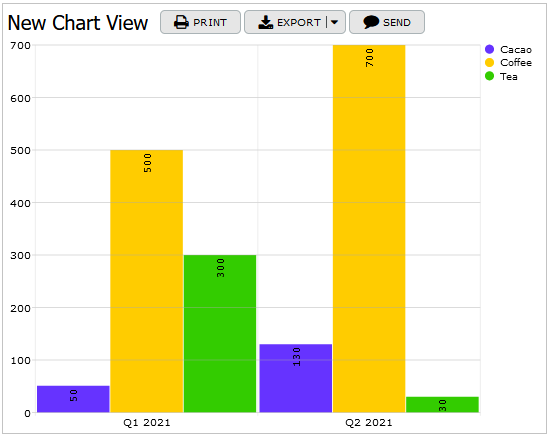# Working with Formulas

In the process of designing a database plan and later during TeamDesk database setup and refinement, you will most likely encounter the need to create something more complex than a simple column that stores user-generated entries. With TeamDesk, you may need the system to calculate or generate data entries automatically based on formulas you create in formula columns and in the default/calculate option of simple physical columns. Additionally, there is a custom formula option in filters of views, buttons, triggers, documents, dashboards, reminders, and notifications.

Formulas can be used for simple cases, such as when you need to sum two numeric values, or for more complicated calculations. In TeamDesk, a formula is a type of rule used to calculate a specific result that can be stored as a column value or used in other ways (for example, a row colorization formula is used to define conditions when rows in the table should be highlighted).

## Example 1

``````List("\n", List(" ",[Street],[Building]), [City], [State], [Zip Code], "USA")
``````

"Duration" column:

``````[End Time]-[Start Time]
``````

"ISO Week Number" column:

``````ISOWeek([Date])
``````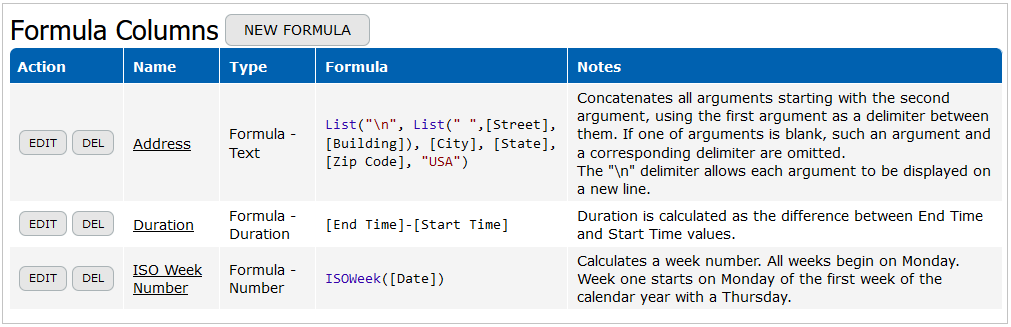You can see the formula columns with calculated results below: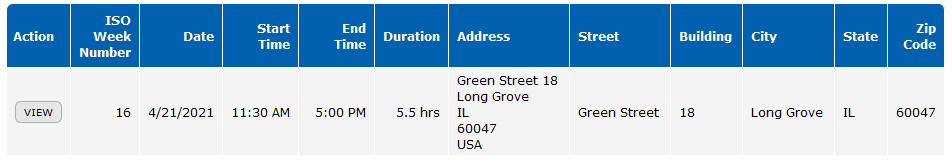## Example 2

Custom Formula in the Filter of a Table View:

``````Ask(Any([Language], Parameter([Language])))
``````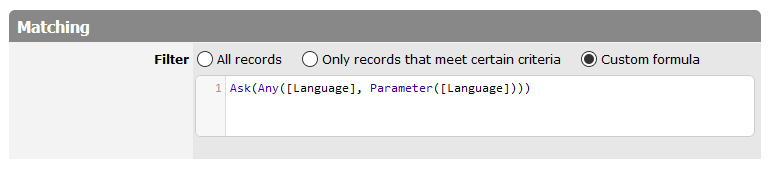The "Language" column is a Text column with the activated multi-choice check boxes option. As a result, if any language selected in the filter is listed in the interpreter profile record, such a record is included in the view.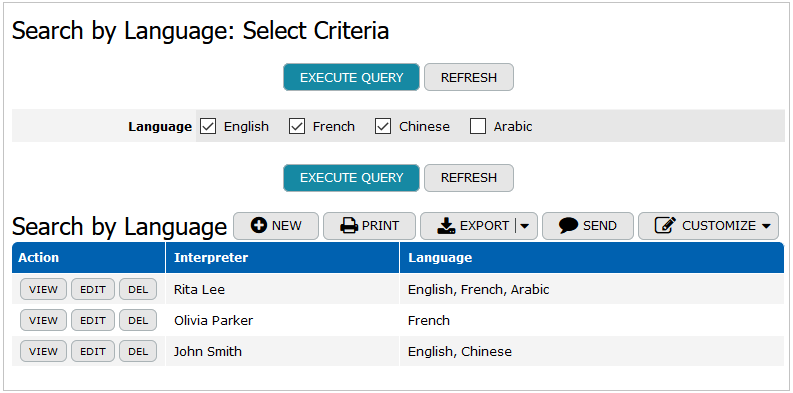## Example 3

Colorization formula in a Chart View:

``````Case([Product], "Cacao", "#6633FF", "Coffee", "#FFCC00", "Tea", "#33CC00")
``````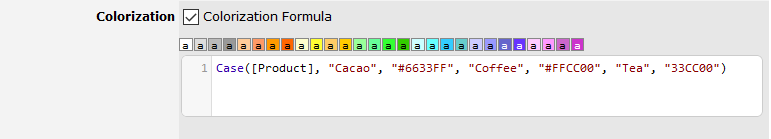As a result, the chart is colored according to the specified colors.